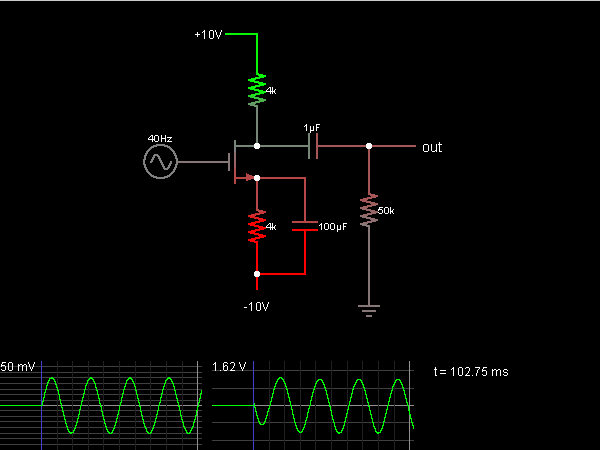# Circuit Simulator - Common-Source Amplifier

## Where can I get Common-Source Amplifier Circuit Diagram with Explanation?

IndiaBIX provides you lots of fully solved Common-Source Amplifier circuit diagram with detailed explanation and working principles.

## How to design a Common-Source Amplifier (electronic circuit)?

You can easily design the Common-Source Amplifier circuit by practicing the exercises given below. Here you can design and simulate your own electronic circuits with this Online Circuit Designer and Simulator.### Circuit Description:

This is a common-source amplifier, which amplifies the input voltage about 30 times.

The gain of this amplifier is determined partly the transconductance of the MOSFET. This depends on the bias point in the circuit; here it averages about 9 mA/V. This means that a change in the gate voltage causes a change in the drain current that is 9 mA/V times the gate voltage change.

The drain current goes through a 4k ohm resistor. The capacitor connected to the source is supposed to act as a short circuit at the input frequency, so it and the source resistor can be ignored. A change in input of 50 mV causes a change in drain voltage of 9 mA/V * 50 mV * 4000 ohms, or about 1.8 V. This means the predicted output gain is 36. Here it is less because the transconductance changes over the input cycle (the amplifier isn't quite linear), we are ignoring the output resistor, and the source capacitor isn't quite a short circuit like we supposed. -- Credits: Mr. Paul Falstad.

 Azad said: (May 8, 2013) Hi, can you more information about it, to any student like come read it can get some information to use it in the discussion of lab experiment. Thanks.